# Compare Two Dates in Excel & Google Sheets

This tutorial will demonstrate how to compare dates in Excel and Google Sheets.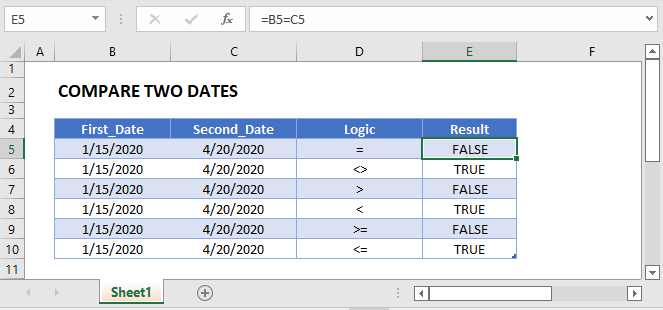## Date Serial Numbers

It’s important to understand how Excel stores dates and times.

Dates are stored as serial numbers; each whole number representing a unique date. 1 represents 1/1/1900 and each number after that represents the days following 1/1/1900.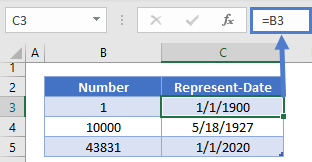Times are stored as decimal values representing a time of a day.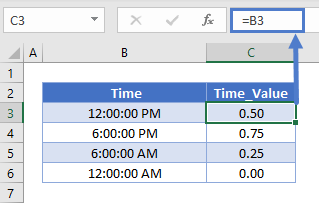A serial number containing a decimal value represents a date and time: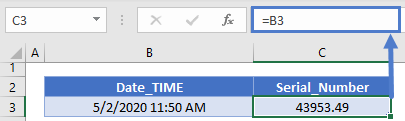## Comparing Two Dates

To compare dates, you can use the usual Excel operators: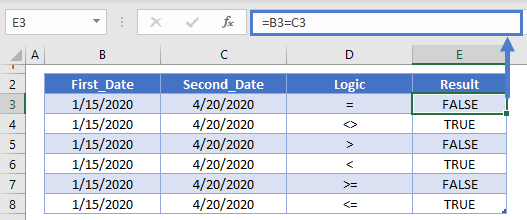### Are Dates Equal?

You can test if dates are equal using the equals (=) sign. (Note: of course this won’t work as desired if the dates contain time values as well. We discuss this more below).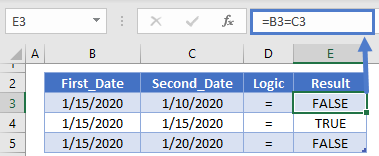### Is Date Greater Than Another Date?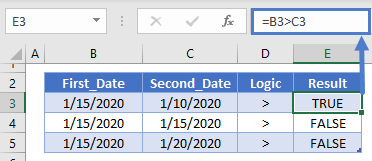### Is Date Less Than or Equal to Another Date?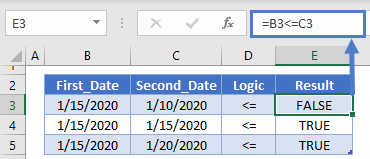## Comparing Two Dates with Times

However, if the dates contain times then you might get unexpected results: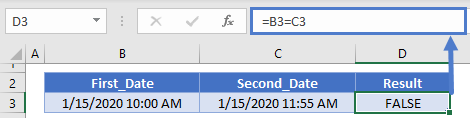Instead, to compare dates only you can use the TRUNC Function to remove the times when making the comparison:

``=TRUNC(B3)=TRUNC(C3)``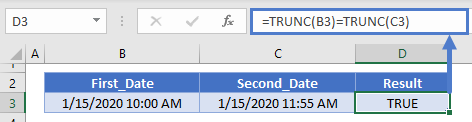### Easy Excel Automation## Compare Today with a Date

You can use the TODAY Function to compare today’s date with another date: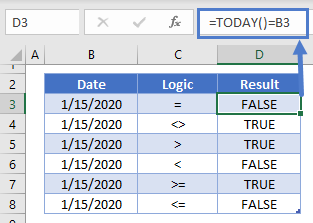## Date Compare & IF Function

In the above examples, we were simply returning TRUE or FALSE depending on the result of the comparison. Instead, we can add the comparisons to an IF Function.  This formula will test if an item is “overdue”, by comparing today’s date with a due date:

``=IF(TODAY()>B3,"Overdue","")``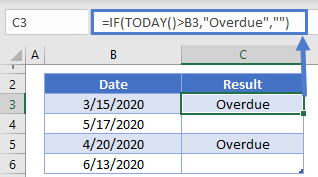## Date Differences

The above examples will tell you if one date is different than another date, but it won’t tell you the amount of difference.

### Days Difference Between Dates

To calculate the number of days between dates simply subtract the dates: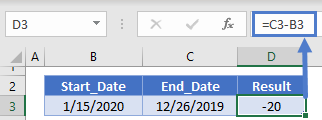### Months or Years Difference Between Dates

To calculate the number of months or years between dates, you’ll need to use the DATEDIF Function:

``=DATEDIF(B3,C3,D3)``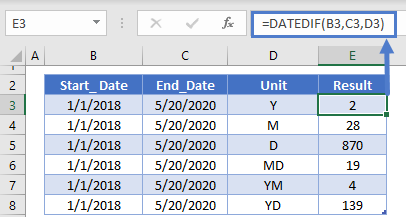## Comparing Dates in Google Sheets

All of the above examples work exactly the same in Google Sheets as in Excel.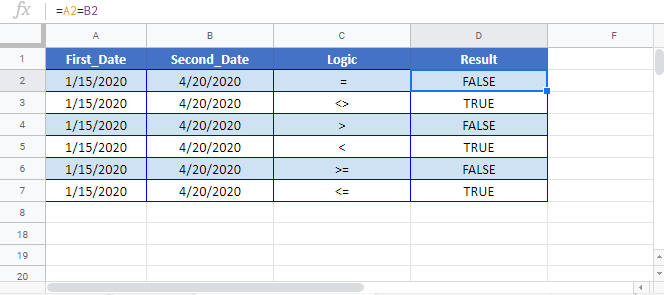### Excel Practice Worksheet

Practice Excel functions and formulas with our 100% free practice worksheets!